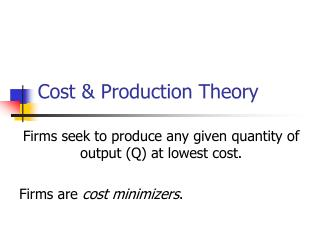Download PresentationCost & Production Theory

Cost & Production Theory - PowerPoint PPT Presentation

Cost & Production Theory. Firms seek to produce any given quantity of output (Q) at lowest cost. Firms are cost minimizers. Costs. C = rK + wL r is the price of capital, w is the wage Cost is the sum of each input quantity multiplied by its price when input prices reflect all costsI am the owner, or an agent authorized to act on behalf of the owner, of the copyrighted work described.
Download PresentationCost & Production Theory

Download Policy: Content on the Website is provided to you AS IS for your information and personal use and may not be sold / licensed / shared on other websites without getting consent from its author.While downloading, if for some reason you are not able to download a presentation, the publisher may have deleted the file from their server.

- - - - - - - - - - - - - - - - - - - - - - - - - - E N D - - - - - - - - - - - - - - - - - - - - - - - - - -
Presentation Transcript
1. Cost & Production Theory Firms seek to produce any given quantity of output (Q) at lowest cost. Firms are cost minimizers.

2. Costs • C = rK + wL • r is the price of capital, w is the wage • Cost is the sum of each input quantity multiplied by its price • when input prices reflect all costs • including opportunity costs

3. Economic Costs are Opportunity Costs • Economic Cost includes both implicit and explicit costs • Explicit Costs – payment to others • Implicit Costs – cost of owned inputs, or other costs that do not generate explicit payments

4. Costs and Output • Long-Run Total Cost or LTC • Combinations of Cost and Output Q • (C1,Q1), (C2,Q2), (C3,Q3) • Long-run average cost • LAC = (LTC/Q) • Long-run marginal cost • LMC = ΔLTC/ΔQ

5. Short-run vs. Long-run • Long-run: all inputs variable • Short-run: one or more inputs fixed • Total Product: Q = f(K0,L) • Average Product of Labor: APL = Q/L • Marginal Product of Labor: MPL = ΔQ/ΔL • Law of diminishing marginal product • As more labor is employed with a fixed amount of capital, labor’s marginal product (MPL) eventually declines

6. Short-run Costs • Total Cost = rK0 + wL = TC • Total Fixed Cost = rK0 = TFC • Total Variable Cost = wL = TVC • TC = TFC + TVC • Average Fixed Cost: AFC = TFC/Q • Average Variable Cost: AVC = TVC/Q • Average Total Cost: ATC = TC/Q

7. Short-run Marginal Cost • SMC = ΔTC/ΔQ • ΔTFC/ΔQ = 0 • SMC = ΔTVC/ΔQ = ΔTC/ΔQ • SMC = AVC at its minimum • SMC = ATC at its minimum

8. Short-run Cost & Product • AVC and MC are inversely related to APL and MPL • MPL > APL implies MC < AVC • Max MPL corresponds to Min MC • MPL = APL implies MC = AVC • MPL < APL implies MC > AVC

9. Short-run and Long-run Cost • Short-run and long-run costs are equal ONLY at a long-run optimum • The quantity where short-run fixed K0 minimizes long run cost • ATC = LAC • Only at the minimum of LAC are all average and marginal costs equal • LAC = LMC = ATC = SMC

10. Economies of Scale • Economies of scale: LAC is decreasing • Costs increase less than proportionately with output • Diseconomies of scale: LAC increasing • Costs increase more than proportionately with output • Constant returns to scale: LAC = LMC • Costs increase exactly in proportion to output • Minimum Efficient Scale or MES • The quantity at which economies of scale end and constant returns begin

11. Economies of Scope • A firm can produce two products together more cheaply than producing each product separately • C(X,Y) < C(X) + C(Y)

12. Estimating Short Run Costs • General Form: TVC = V(Q, P, K) • Q = Output quantity • P = price of variable input(s) • K = quantity of capital or fixed input(s)

13. Linear Short Run Costs • Linear: TVC = a + bQ • MC=b, a constant • AVC declines as Q increases • Cannot represent • U-shaped AVC • Upward sloping MC

14. Cubic Short Run Costs • Cubic: TVC = aQ + bQ2 + cQ3 • AVC = a + bQ + cQ2 • For U-shaped AVC: a > 0, b < 0, c > 0 • MC = a + 2bQ + 3cQ2 • Can be upward sloping • Q for minimum AVC: Qmin = -b/(2c)

15. Cost Project COST REGRESSION PROJECT Econ 4570 DATA: TVACostData.xls under Cost Project Data on D2L • The data file contains actual cost and output data for TVA non-hydroelectric plants in 2003. Use this data file to run a linear regression as specified below. Have the computer program plot the actual vs. predicted values of the dependent variable (in Excel, check the box marked “Line Fit Plots”). Dependent Variable: AVC Explanatory Variables: Q, QSQ. a. Which coefficients (including the constant) are statistically significant at the 10% level or better? Which are not significant? b. How much of the variation in the dependent variable is explained by the estimated equation? c. Is the equation as a whole statistically significant? At what level? d. Is the estimated average variable cost curve U-shaped? Why?